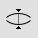# CrvDeviationAnalyze

Curve >

Deviation

The CrvDeviation command reports the maximum and minimum distances between two curves.

#### Steps

1. Select two curves.
2. The command may find one or more intervals of overlap between the two curves, or it may report that the curves do not overlap.

For each overlap interval, three pairs of indicator marks with indicator lines between them will appear, joining the ends of the overlap interval to the points of greatest deviation within the interval.

Minimum deviation = Green Marks

Maximum deviation = Red Marks

• For each overlap interval, the three deviation distances are reported at the command line, along with the world coordinates of the interval ends on each curve.

• Use the Command History window or press the F2key to view the data in a separate window.

3. Press Enter to turn off the deviation display.
Command-line options

Type subcrv to select part of a curve as input.

• For each overlap interval, the three deviation distances are reported at the command line, along with the world coordinates of the interval ends on each curve.
• Minimum deviation will be 0 when the two curves intersect.